## 66) Equation

Bonds of 11 to 20: Building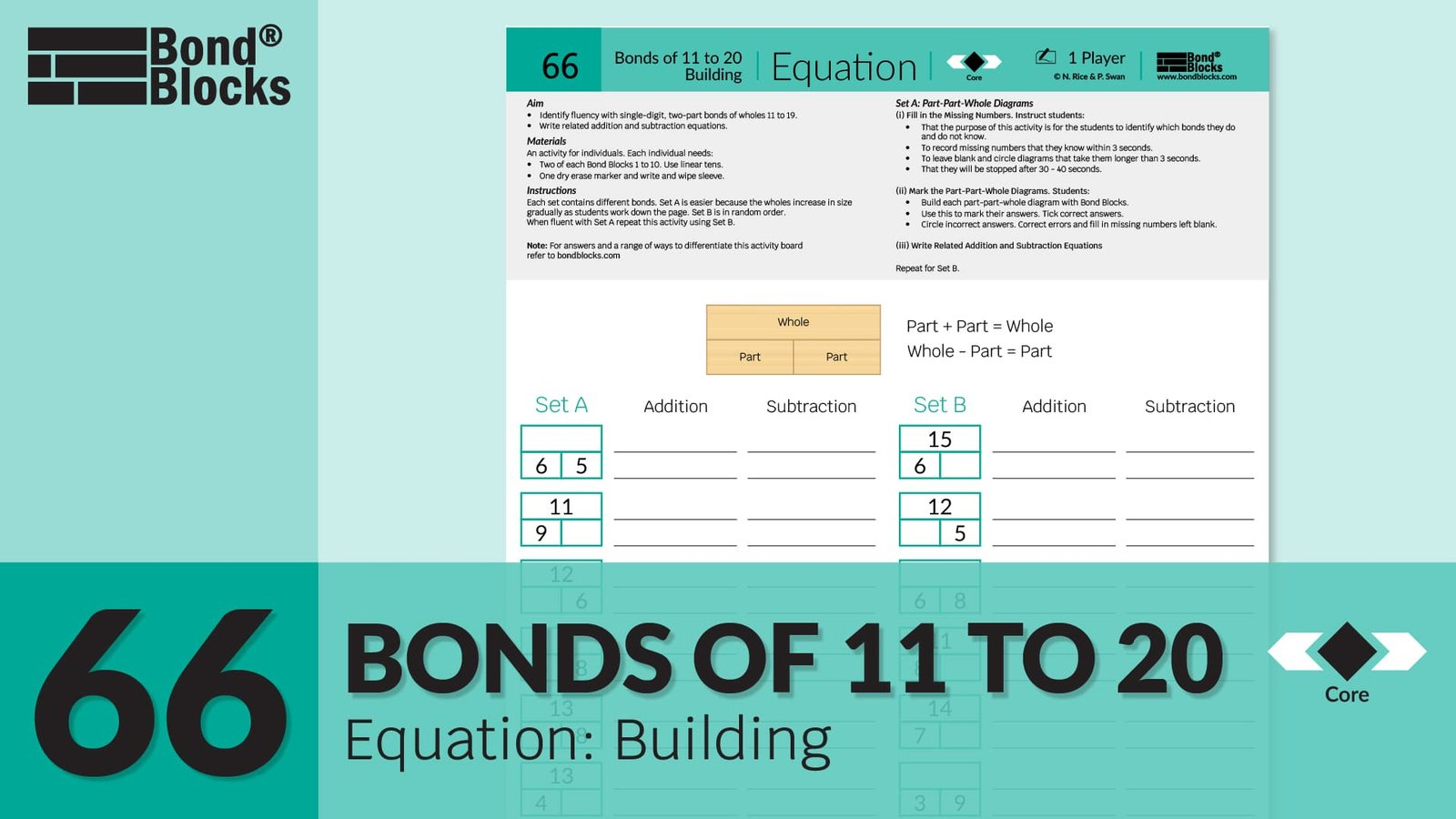### Mathematics

Apply the concept of related addition and subtraction equations, rearranged using the part-part-whole relationship, to find a missing part or whole.

Develop fluency recalling single-digit, two-part bonds of wholes 11 to 19.
(Where the part is greater than one and the commutative property is applied.)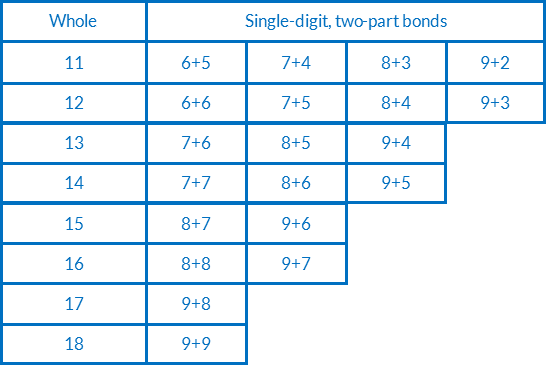This Equation Building activity combines Missing Number concepts. It is abstract, using only numbers, and assumes students have completed the Equation Building and Missing Number activities in previous chapters.

### Language

• part-part-whole: where the two parts are a bond of the whole number
• missing (unknown) part
•  missing (unknown) whole
• subtract
• equals

### Core Activity Support MaterialsClick to open in a new tab.## Differentiation

### A little easier

##### Use Bond Blocks

Use Bond Blocks to identify the missing number by representing both parts and the whole.

For example, to check a missing partFor example, to calculate a missing part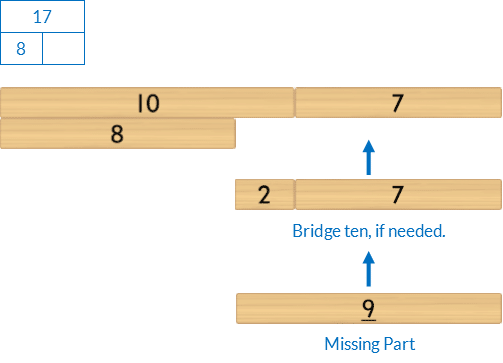For example, to check a missing whole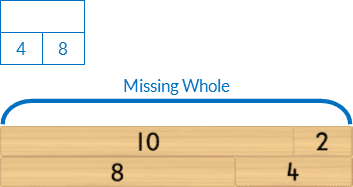##### Part-Part-Whole diagrams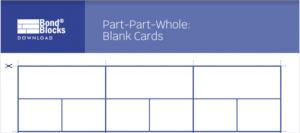Students fill them in for the specific bonds they identified as still needing to develop fluency with.

• Cover different numbers to practice recall.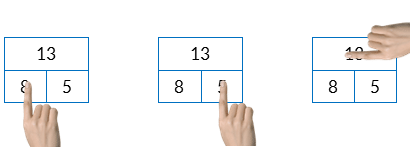##### Fluency cards

Each of the single-digit, two-part bonds for wholes 11 to 18 can be downloaded and printed as Addition and Subtraction Cards. Select cards for a specified bond/s and use these to play Memory, Go Fish, Snap where the goal is to collect related addition and subtraction equations for each whole.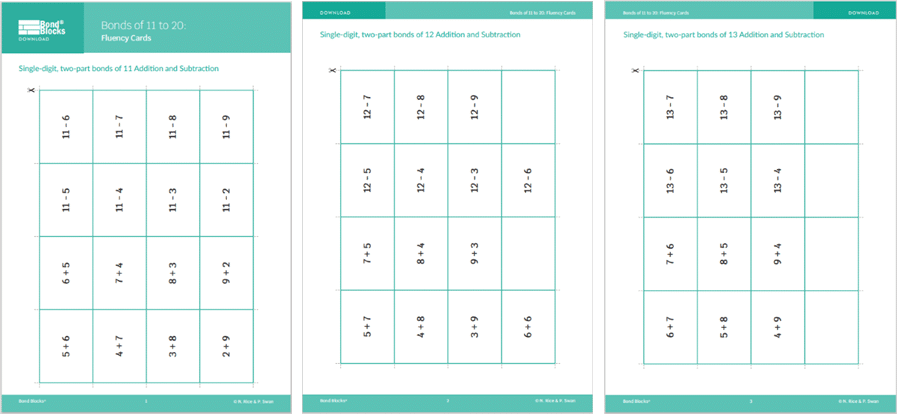### A little harder

##### Atypical arrangement

Write equations in an atypical order.

Whole = Part + Part

Part = Whole – Part##### Equation building for wholes in the twenties

Use Bond Blocks to represent part-part-whole for wholes in the twenty. Make related addition and subtraction equations. Then change these equations to missing number equations for other students to solve.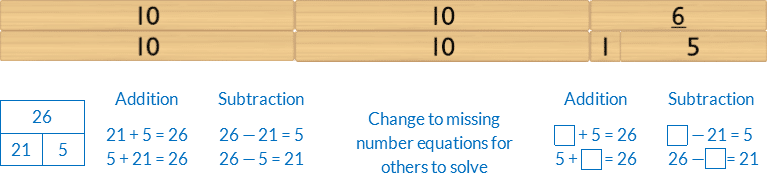The same whole can be partitioned differently to create new equations.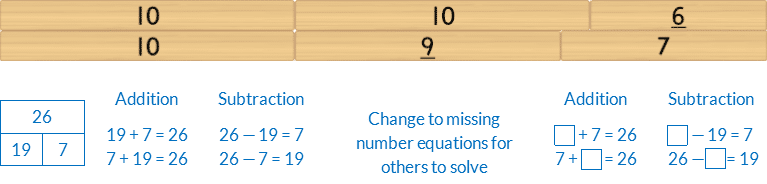Look for patterns in how these two different partitions are related.

• Which numbers increased/decreased? By how much? Why?
• Which numbers stayed the same?
• What would happen if the 19 was changed to 17? Why? Explain this using Bond Blocks

### Progression

In the next activity, students apply the missing number concept to missing number addition and subtraction equations. Go to

##### Activity 67

Bonds of 11 to 20: Missing Number Equations, Racing Motorcycles